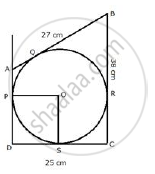Share

# In Quadrilateral Abcd; Angles D = 90°, Bc = 38 Cm and Dc = 25 Cm. a Circle is Inscribed in this Quadrilateral Which Touches Ab at Point Q Such that Qb = 27 Cm, Find the Radius of the Circle. - Mathematics

Course

#### Question

In quadrilateral ABCD; angles D = 90°, BC = 38 cm and DC = 25 cm. A circle is inscribed in this quadrilateral which touches AB at point Q such that QB = 27 cm, Find the radius of the circle.

#### SolutionBQ and BR are the tangents from B to the circle.
Therefore, BR =BQ = 27 cm.
Also RC = (38 −; 27) = 11cm
Since CR and CS are the tangents from C to the circle
Therefore, CS = CR = 11 cm
So, DS = (25 − 11) = 14 cm
Now DS and DP are the tangents to the circle
Therefore, DS = DP
Now, ∠PDS = 90° (given)
and OP  ⊥ AD, OS ⊥  DC
therefore, radius = DS = 14 cm

Is there an error in this question or solution?